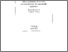# On a Problem of Source Reconstruction for Parabolic Equation

Samarskaya, E.A. & Tishkin, V. (1996). On a Problem of Source Reconstruction for Parabolic Equation. IIASA Working Paper. IIASA, Laxenburg, Austria: WP-96-038Preview Text WP-96-038.pdf Download (411kB) | Preview

## Abstract

In the present paper the problem of reconstruction of the right-hand side of an advection-diffusion equation is considered. This type of equation is used in many models of contamination transport in domains such as air, groundwater and surface water. Using the method of conjugate equations, one can reduce the problem to an integral equation of the first kind. In the paper a discrete analog of this integral equation is constructed on the basis of discretization of the initial advection-diffusion equation and the usage of the conjugate equation technique. For solving the obtained discrete analog of the integral equation Tikhonov's method of regularization is applied. The parameter of regularization is chosen in accordance with the residual principle. Series of numerical calculations show efficiency of the method.

Item Type: Monograph (IIASA Working Paper) Dynamic Systems (DYN) IIASA Import 15 Jan 2016 02:08 27 Aug 2021 17:15 https://pure.iiasa.ac.at/4988View Item